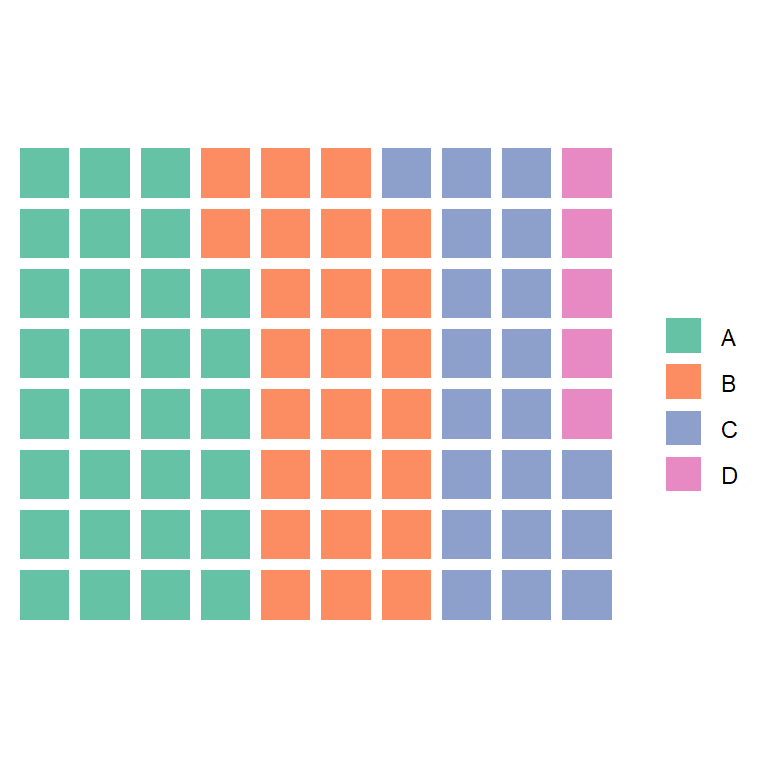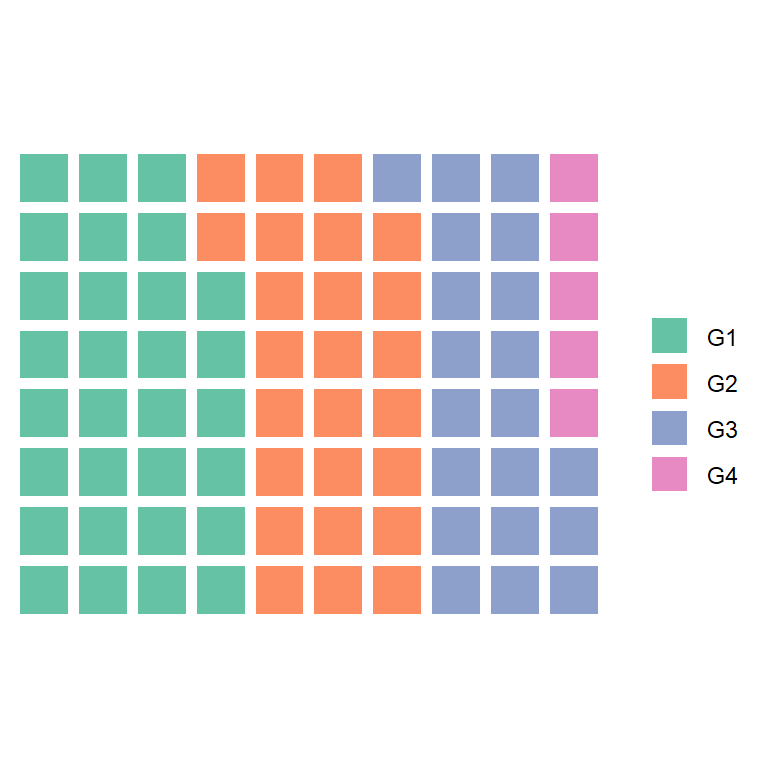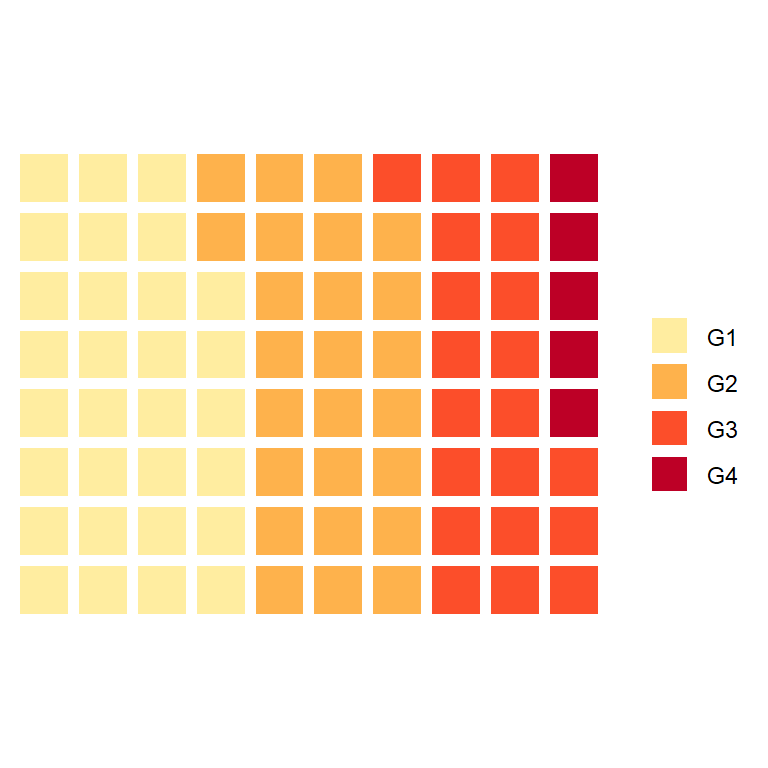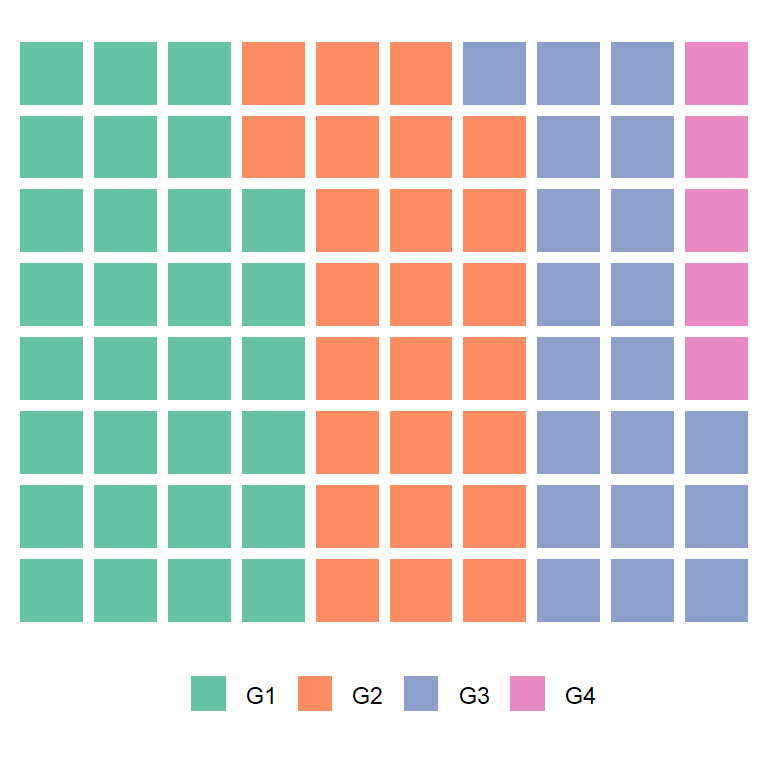# Waffle chart (square pie) in ggplot2

Bob Rudis

## Most basic waffle chart

The `waffle` package function contains a function of the same name that can be used to create waffle charts, also known as square pie charts or gridplots, based on ggplot2.

To create a basic waffle plot pass a vector containing the count for each group to the function. The number of rows of the plot can be selected with `rows` (defaults to 10). Choose a value according to your data.

``````# install.packages("waffle", repos = "https://cinc.rud.is")
library(waffle)

# Vector
x <- c(30, 25, 20, 5)

# Waffle chart
waffle(x, rows = 8)``````Use a named vector to change the legend names

If you name the variables, the legend will display their names.

``````# install.packages("waffle", repos = "https://cinc.rud.is")
library(waffle)

# Vector
x <- c(G1 = 30, G2 = 25, G3 = 20, G4 = 5)

# Waffle chart
waffle(x, rows = 8)``````Color customization

You can pass a vector of colors to the `colors` argument. Pass as many colors as the number of components in the input vector.

``````# install.packages("waffle", repos = "https://cinc.rud.is")
library(waffle)

# Vector
x <- c(G1 = 30, G2 = 25, G3 = 20, G4 = 5)

# Waffle chart
waffle(x, rows = 8,
colors = c("#FFEDA0", "#FEB24C", "#FC4E2A", "#BD0026"))``````Legend position

The legend position can be changed with `legend_pos` argument. Possible values are `"right"` (default), `"bottom"`, `"left"`, `"top"` and `"none"` to remove the legend.

``````# install.packages("waffle", repos = "https://cinc.rud.is")
library(waffle)

# Vector
x <- c(G1 = 30,  G2 = 25, G3 = 20, G4 = 5)

# Waffle chart
waffle(x, rows = 8,
legend_pos = "bottom")``````In case you want to increase the margin of the plot respect to the legend pass a numeric value to `pad` argument (defaults to 0).